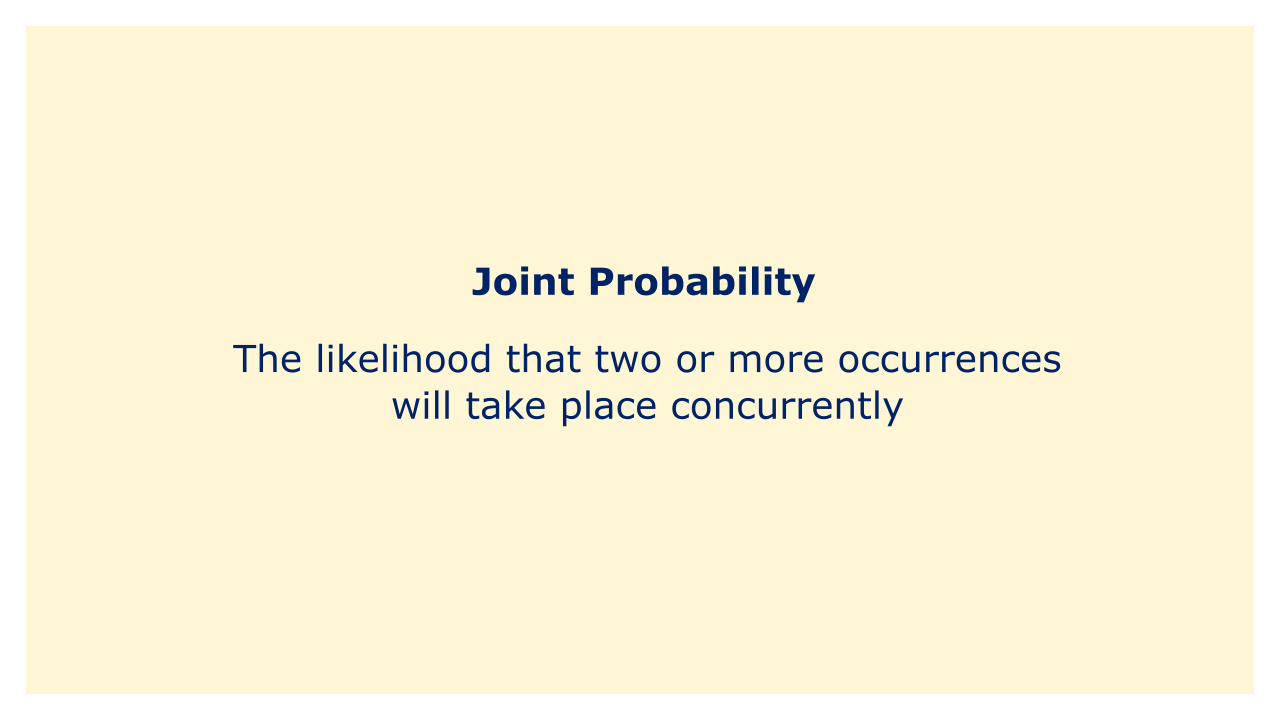# Joint ProbabilityImage: Moneybestpal.com

### Joint probability, as used in probability theory, is the likelihood that two or more occurrences will take place concurrently. It assesses the probability that two or more random variables will all take particular values simultaneously.

Joint probability is a term used frequently in finance to describe the possibility that two or more events will occur simultaneously.

The product of the individual probabilities of each event occurring, assuming that the other event has already occurred, yields the joint probability of two events A and B indicated as P(A and B) or P(A, B). This can be stated as:

P(A and B) = P(A) * P(B|A)

where P(B|A) is the conditional probability of event B occurring given that event A has occurred and P(A) is the likelihood of event A occurring.

For illustration, suppose we want to determine the combined likelihood that Stock A and Stock B would appreciate in value. We have two stocks, Stock A and Stock B. Given that Stock A has already gained in value and that there is a 0.6 chance of Stock A increasing in value and a 0.5 chance of Stock B increasing in value, the combined likelihood that both stocks will rise in value is:

P(A and B) = P(A) * P(B|A) = 0.6 * 0.5 = 0.3

Hence, there is a 30% possibility that the value of both stocks will rise at the same moment.

It is also possible to determine the correlation between two variables using joint probability. Two variables are said to be independent if the product of their marginal probabilities equals the joint probability of the two variables. The variables are said to be dependent, though, if the joint probability does not equal the marginal probabilities' product.
Tags